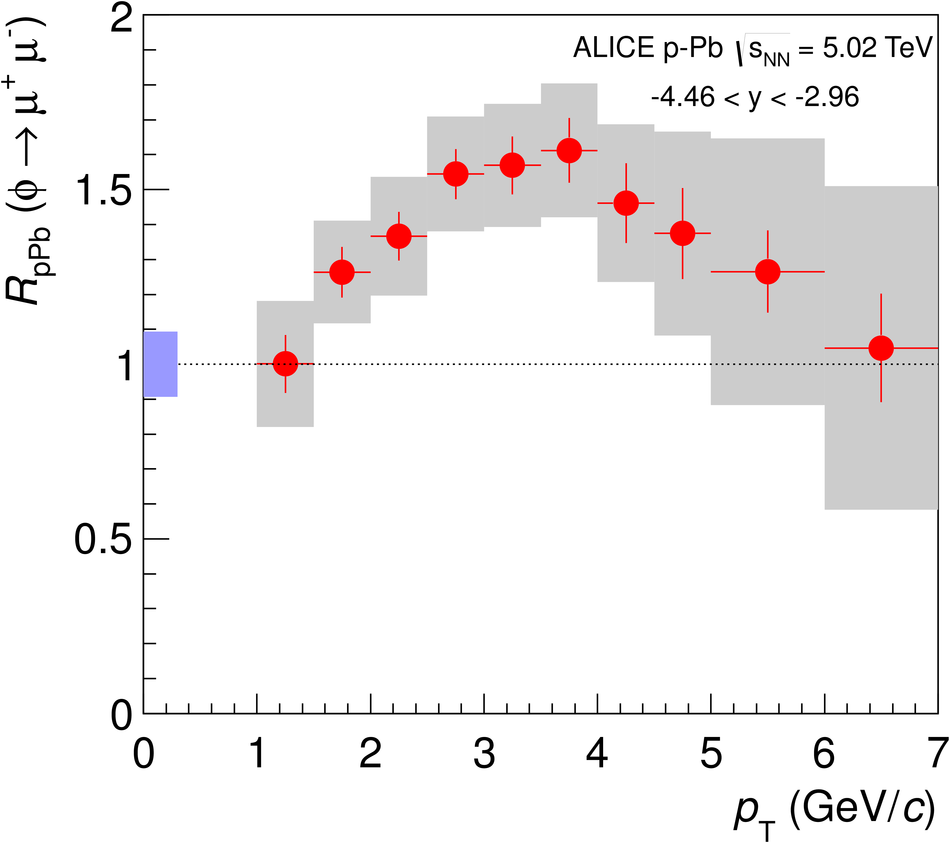# $φ$-meson production at forward rapidity in p-Pb collisions at $\sqrt{s_{\rm NN}}$ = 5.02 TeV and in pp collisions at $\sqrt{s}$ = 2.76 TeV

The first study of $\phi$-meson production in p-Pb collisions at forward and backward rapidity, at a nucleon-nucleon centre-of-mass energy $\sqrt{s_{\rm NN}} = 5.02$ TeV, has been performed with the ALICE apparatus at the LHC. The $\phi$-mesons have been identified in the dimuon decay channel in the transverse momentum ($p_{\rm T}$) range $1 < p_{\rm T} < 7$ GeV/$c$, both in the p-going ($2.03 < y < 3.53$) and the Pb-going ($-4.46 < y < -2.96$) directions, where $y$ stands for the rapidity in the nucleon-nucleon centre-of-mass, the integrated luminosity amounting to $5.01 \pm 0.19$ nb$^{-1}$ and $5.81 \pm 0.20$ nb$^{-1}$, respectively, for the two data samples. Differential cross sections as a function of transverse momentum and rapidity are presented. The forward-backward ratio for $\phi$-meson production is measured for $2.96< |y|< 3.53$, resulting in a ratio $\sim 0.5$ with no significant $p_{\rm T}$ dependence within the uncertainties. The $p_{\rm T}$ dependence of the $\phi$ nuclear modification factor $R_{\rm pPb}$ exhibits an enhancement up to a factor 1.6 at $p_{\rm T}$ = 3-4 GeV/$c$ in the Pb-going direction. The $p_{\rm T}$ dependence of the $\phi$-meson cross section in pp collisions at $\sqrt{s}$ = 2.76 TeV, which is used to determine a reference for the p-Pb results, is also presented here for $1 < p_{\rm T} < 5$ GeV/$c$ and $2.5 < y < 4$ for a $78 \pm 3$ nb$^{-1}$ integrated luminosity sample.

Figures

## Figure 1

 Dimuon mass spectrum after combinatorial background subtraction: $\pt$-integrated pp sample (top panels) and $\pt$-integrated p-Pb sample in the backward (centre panels) and forward (bottom panels) rapidity regions, compared to the result of the hadronic-cocktail and the empirical-function fits (left- and right-column panels, respectively). Error boxes on data points (well visible only in some regions on the plots) represent the systematic uncertainty due to the combinatorial background subtraction, while error bars account for the statistical uncertainty. The width of the hadronic-cocktail fit result (red band) combines the statistical uncertainties of the free fit parameters with the systematic uncertainties on the fixed parameters (see text).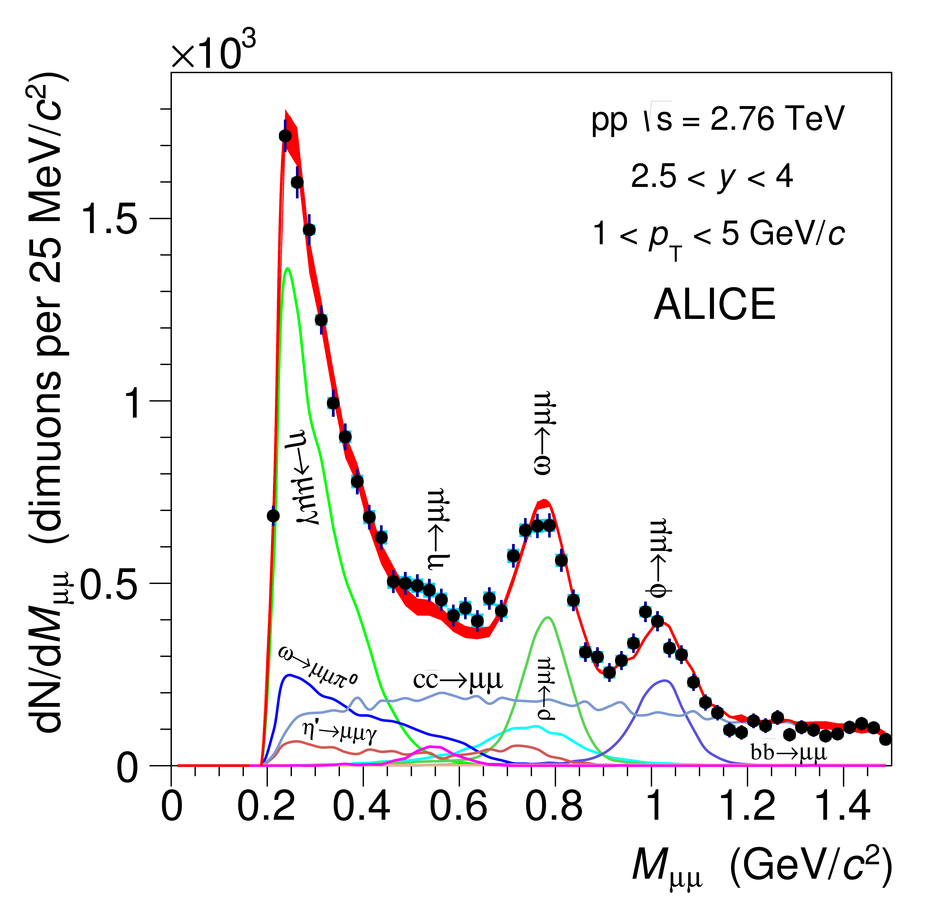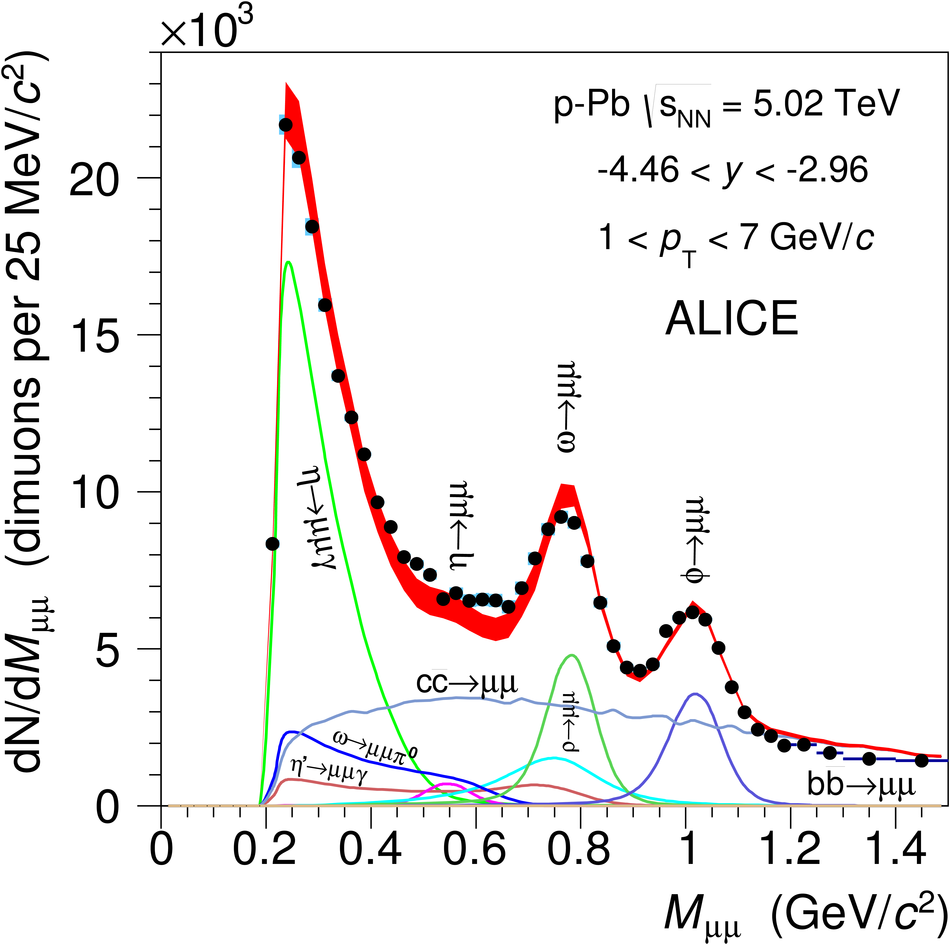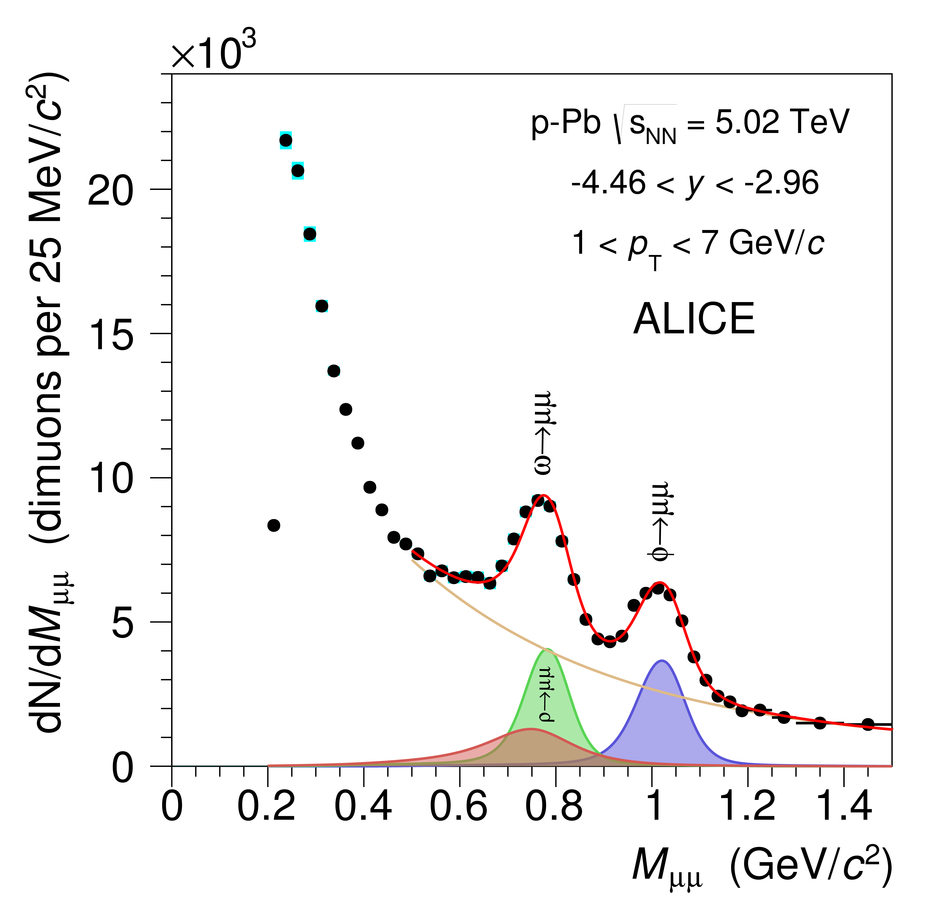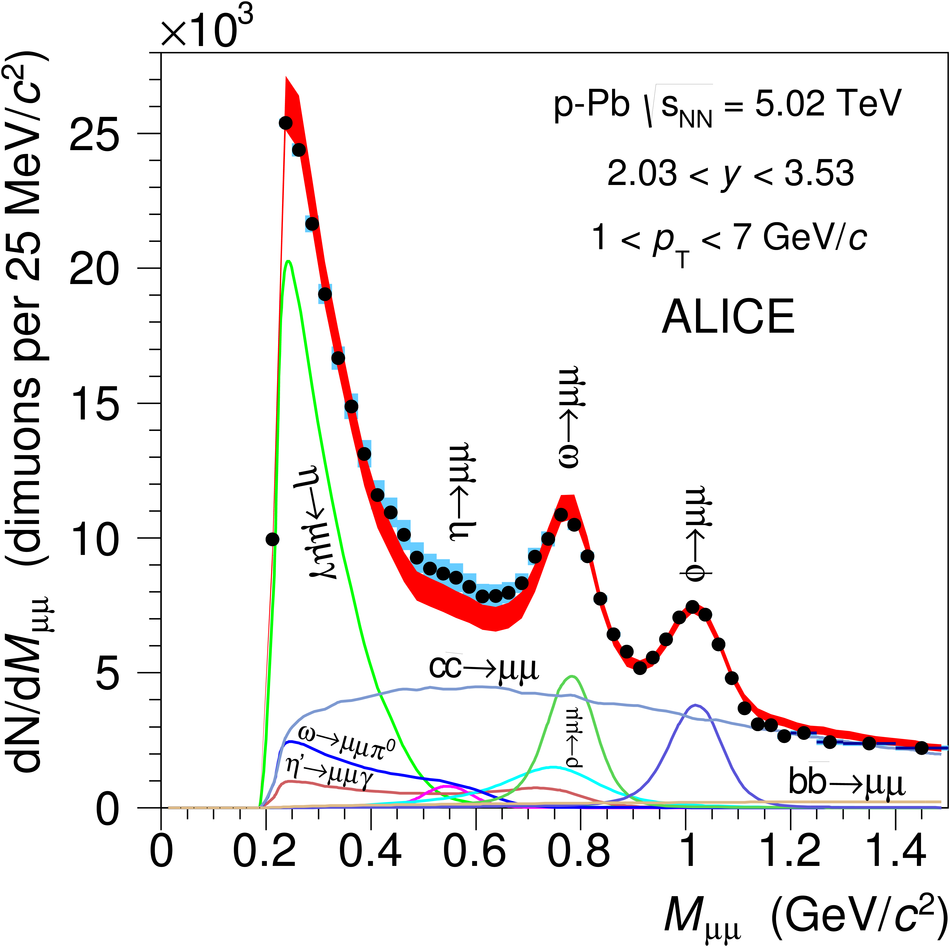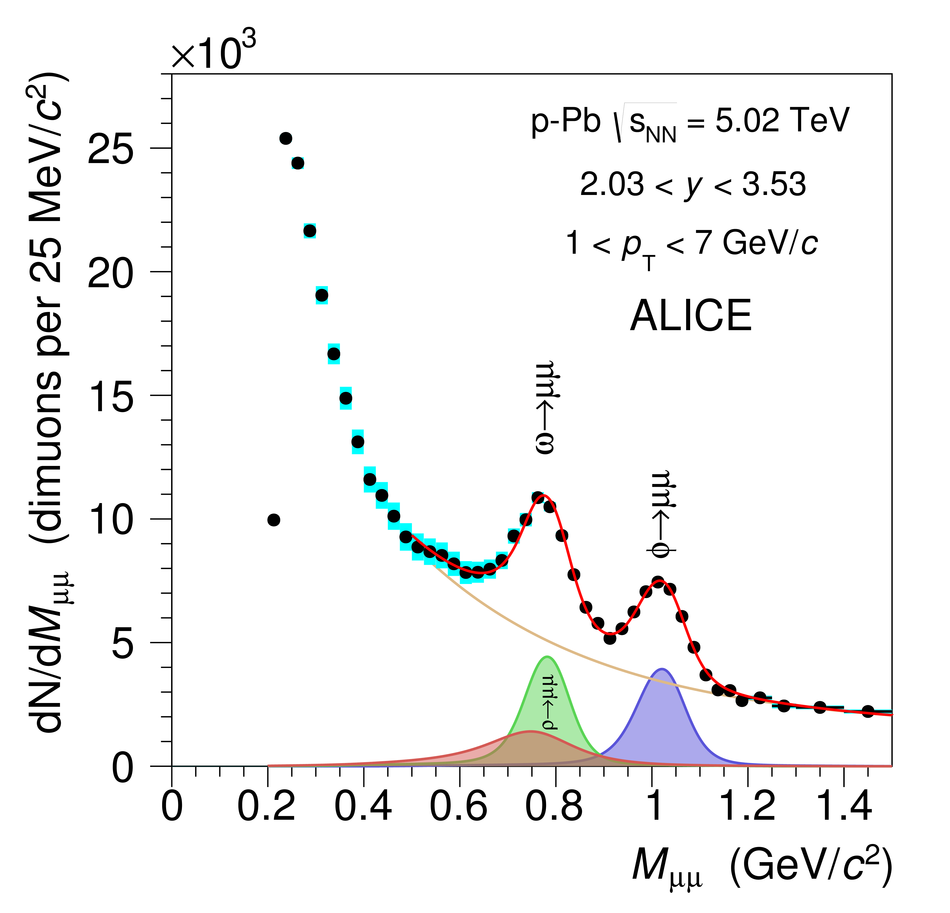## Figure 2

 $\phi$-meson cross section as a function of $\pt$ in pp collisions at $\sqrt{s} = 2.76$ TeV. Error bars and boxes represent statistical and systematic uncertainties, respectively. Predictions from PHOJET and the PYTHIA tunes ATLAS-CSC, D6T, Perugia0 and Perugia11 are also shown for comparison, as well as the result of a fit with the Levy-Tsallis function defined by Eq. (1).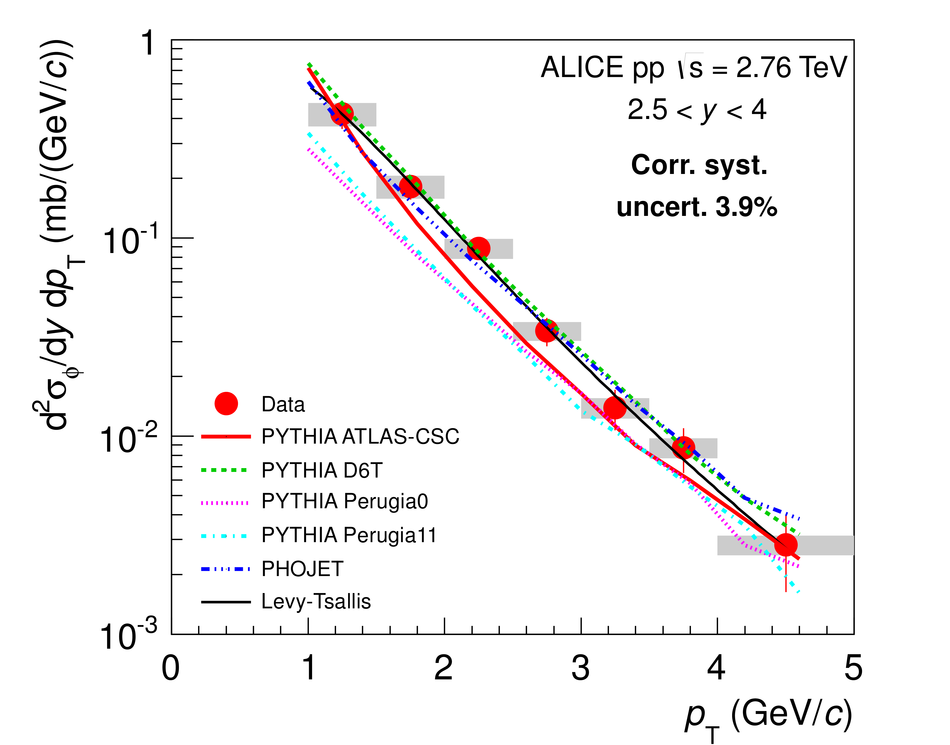## Figure 3

 $\phi$-meson cross section in p-Pb collisions at $\snn = 5.02$ TeV as a function of $\pt$ in the backward (left) and forward (right) rapidity regions. Error bars (smaller than the markers) and boxes represent statistical and systematic uncertainties, respectively. Predictions by HIJING and DPMJET are also shown, together with the result of a fit with the Levy-Tsallis function (Eq. 1).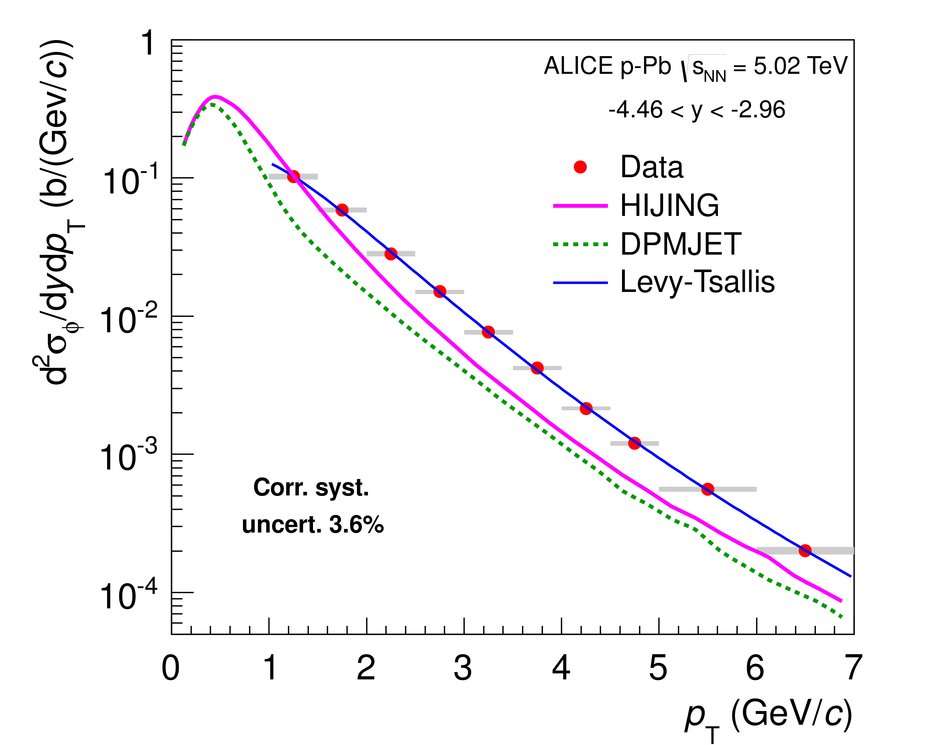## Figure 4

 $\phi$-meson cross section in p-Pb collisions at $\snn = 5.02$ TeV as a function of rapidity, integrated over the range $1 < \pt < 7$ GeV/$c$. Error bars and boxes represent statistical and systematic uncertainties, respectively. Predictions by HIJING and DPMJET are also shown, together with the mid-rapidity data point from the $\phi$-meson measurement in the K$^+$K$^-$ channel, also evaluated in the range $1 < \pt < 7$ GeV/$c$.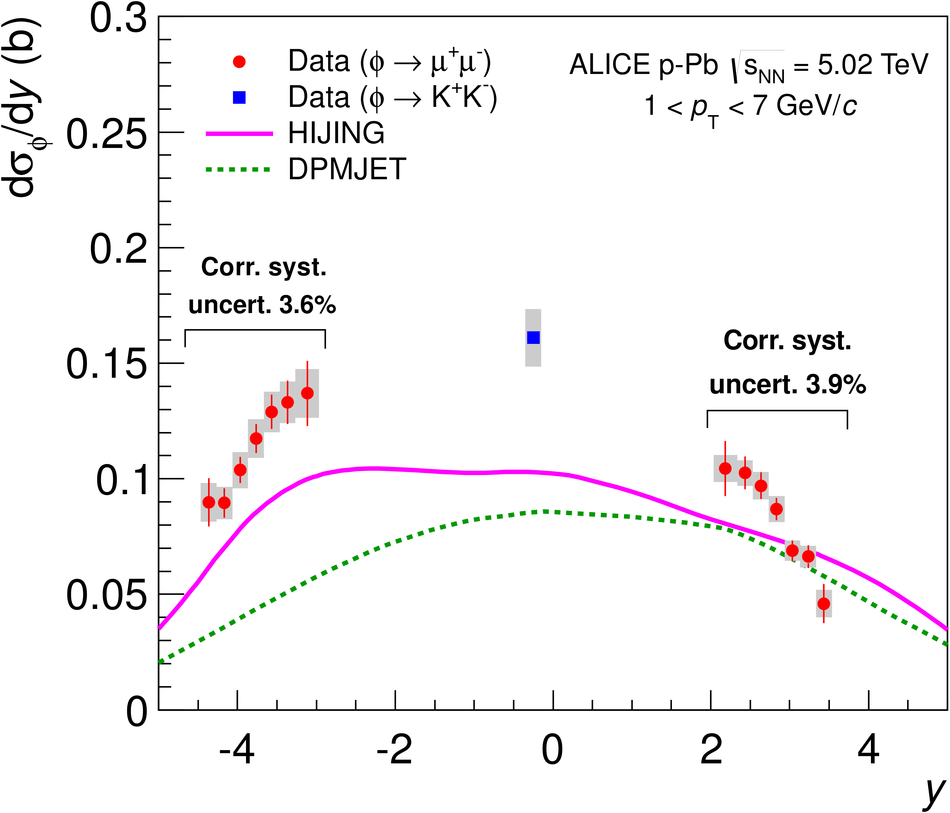## Figure 5

 Forward-backward ratio for the $\phi$-meson in p-Pb collisions at $\snn = 5.02$ TeV as a function of $\pt$, in the rapidity range $2.96 < |y| < 3.53$ common to the two rapidity regions considered in the analysis. Error bars and boxes represent statistical and systematic uncertainties, respectively. The blue box on the left represents the bin-to-bin correlated systematic uncertainty, see Table 7. Predictions from HIJING and DPMJET are also shown for comparison.## Figure 6

 Nuclear modification factor $\RpPb$ in p-Pb collisions at $\snn = 5.02$ TeV for the $\phi$-meson as a function of $\pt$, in the backward (left) and forward (right) rapidity regions considered in the analysis. Error bars and boxes represent statistical and bin-to-bin uncorrelated systematic uncertainties, respectively. The blue box on the left represents the bin-to-bin correlated systematic uncertainty, see Table 7.